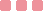# Subtraction Balance Equations GameGame description## Friv Subtraction Balance Equations Game

Friv Subtraction Balance Equations Game is now available to play at Friv for School Game. This is an educational game based on math. In this math game, the player needs to solve the subtraction questions in a different way. There is an equation which is complete on one side and on the other hand you will see an incomplete equation with an empty box. Fill the empty box with the correct answer to earn points. To solve the question, find out which number make the equation true? Cross Out Method Subtraction Game is also available here. Play in mute mode.

Controls: Keyboard.